## Online Education for RS Aggarwal Class 9 Solutions Chapter 13 Volume and Surface Area Ex 13D

These Solutions are part of Online Education RS Aggarwal Solutions Class 9. Here we have given RS Aggarwal Class 9 Solutions Chapter 13 Volume and Surface Area Ex 13D.

Other Exercises

Question 1.
Solution:
(i) Radius of sphere = 3.5cm
(a) Volume = $$\frac { 4 }{ 3 }$$ πr3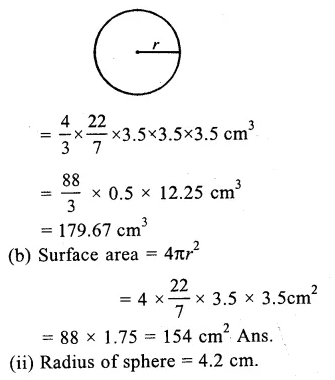Question 2.
Solution:
Let r be the radius of the sphere and volume = 38808 cm3
∴$$\frac { 4 }{ 3 }$$ πr3 = 38803
=> $$\frac { 4 }{ 3 }$$ x $$\frac { 22 }{ 7 }$$ r3 = 38803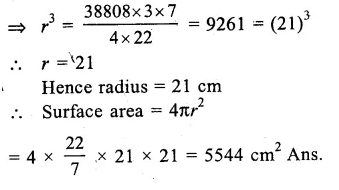Question 3.
Solution:
Let r be the radius of the sphere
∴ Volume = $$\frac { 4 }{ 3 }$$ πr3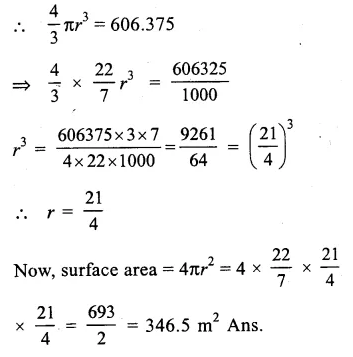Question 4.
Solution:
Surface area of a sphere = 394.24 m2
Let r be the radius, then 4πr2 = 394.24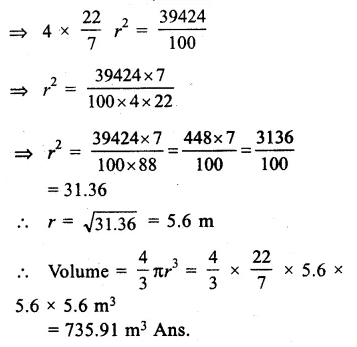Question 5.
Solution:
Surface area of sphere = 576π cm2
Let r be the radius, then 4r2 = 576π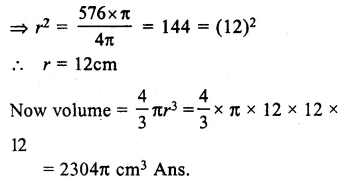Question 6.
Solution:
Outer diameter of shell = 12cm,
Outer radius (R) = $$\frac { 12 }{ 2 }$$ = 6cm
and inner diameter = 8cm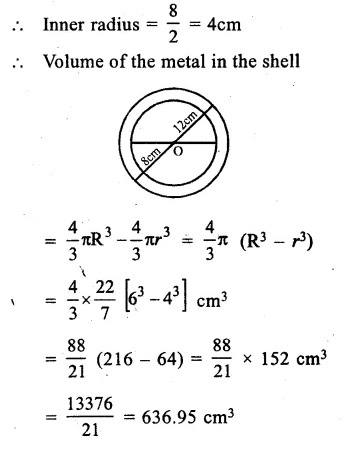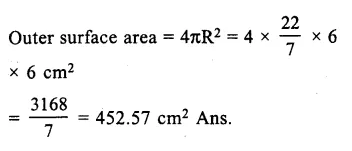Question 7.
Solution:
Length of cuboid of (l) = 12cm
and height (h) = 9cm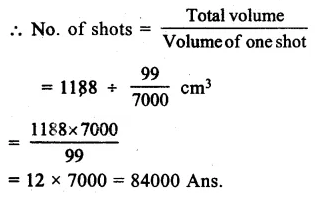Question 8.
Solution:
Radius of sphere (r) = 8cm
Volume = $$\frac { 4 }{ 3 }$$πr3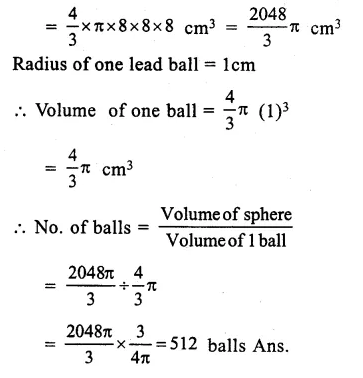Question 9.
Solution:
Radius of solid sphere (R) = 3cm.
Volume = $$\frac { 4 }{ 3 }$$π(R)3 = $$\frac { 4 }{ 3 }$$π(3)3 cm3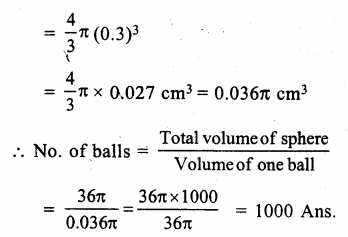Question 10.
Solution:
Radius of metallic sphere (R) = 10.5cmQuestion 11.
Solution:
Diameter of a cylinder = 8cm
Radius (r) = $$\frac { 8 }{ 2 }$$ = 4cm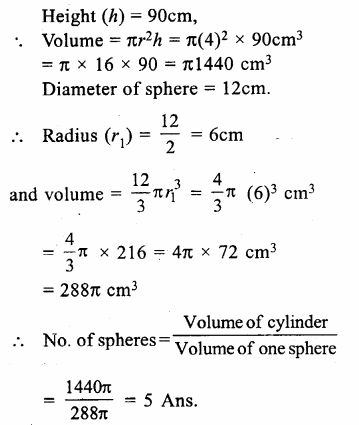Question 12.
Solution:
Diameter of sphere = 6cm
Radius (R) = $$\frac { 6 }{ 2 }$$ = 3cm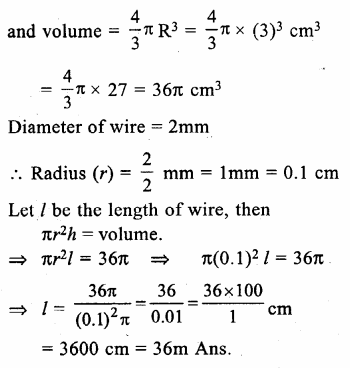Question 13.
Solution:
Diameter of sphere = 18cm
Radius (R) = $$\frac { 18 }{ 2 }$$ = 9cm.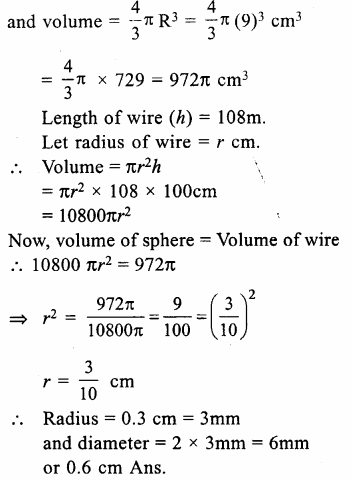Question 14.
Solution:
Diameter of the sphere = 15.6 cm
Radius (R) = $$\frac { 15.6 }{ 2 }$$ = 7.8 cm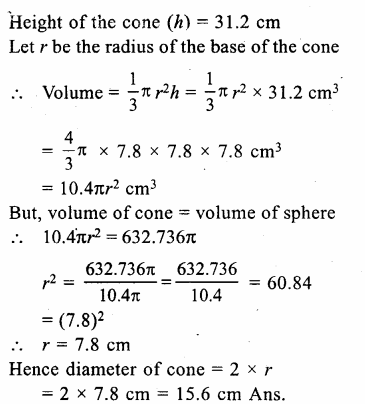Question 15.
Solution:
Diameter of the canonball = 28cm
Radius (R) = $$\frac { 28 }{ 2 }$$ = 14 cm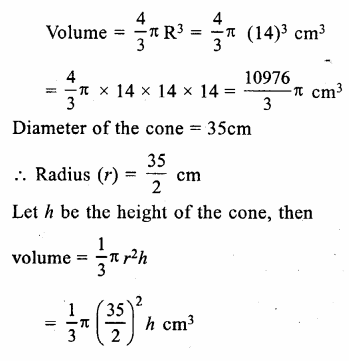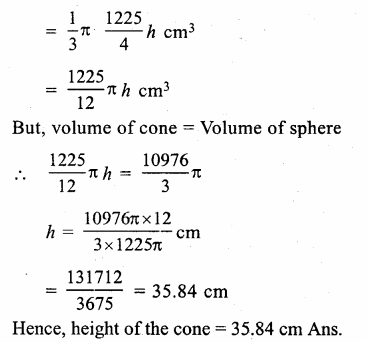Question 16.
Solution:
Given,
Radius of spherical big ball (R) = 3cm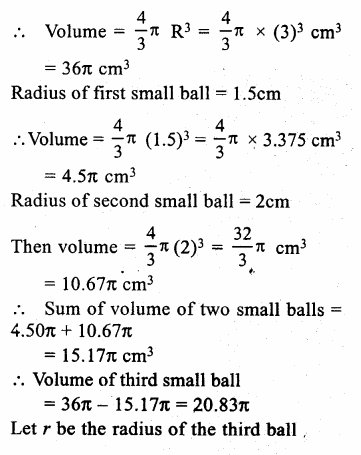Question 17.
Solution:
Ratio in the radii of two spheres = 1:2
Let radius of smaller sphere = r then,
radius of bigger sphere = 2r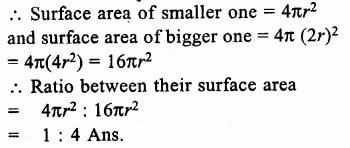Question 18.
Solution:
Let r1 and r2 be the radii of two spheres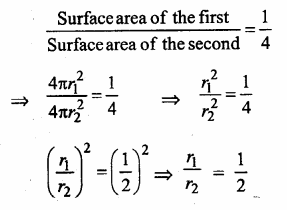Question 19.
Solution:
Radius of the cylindrical tub = 12cm.
First level of water = 20cm
Raised water level = 6.75cm.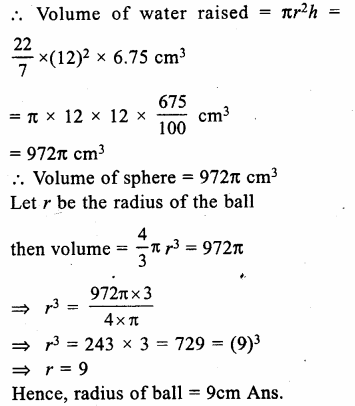Question 20.
Solution:
Radius of the ball (r) = 9cm.
Volume of ball = $$\frac { 4 }{ 3 }$$πr³Question 21.
Solution:
Given,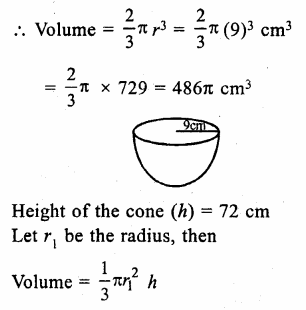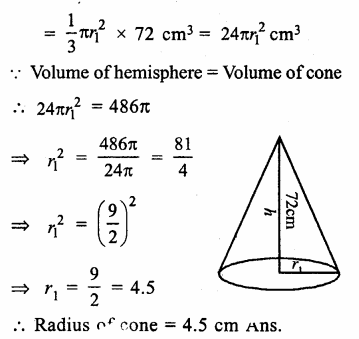Question 22.
Solution:
Given,
Radius of hemispherical bowl (r) = 9cm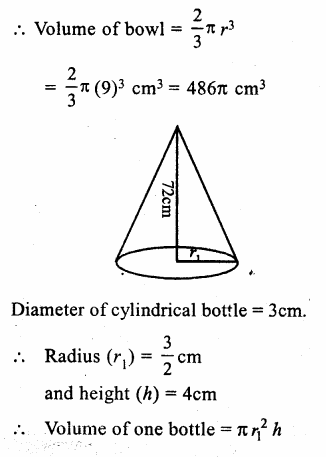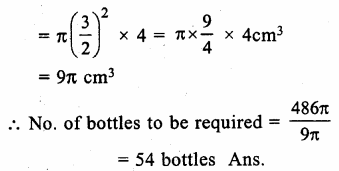Question 23.
Solution:
External radius of spherical shell (R) = 9cm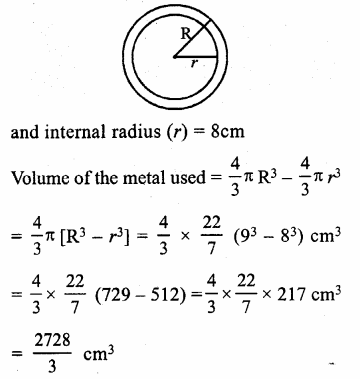Question 24.
Solution:
Inner radius (r) = 4 cm
Thickness of steel used = 0.5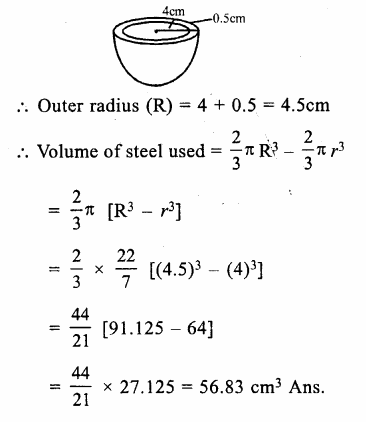Hope given RS Aggarwal Class 9 Solutions Chapter 13 Volume and Surface Area Ex 13D are helpful to complete your math homework.

If you have any doubts, please comment below. Learn Insta try to provide online math tutoring for you.